# 计算机中存储体系的设计

## 概述

$$N_2$$是对$$M_2$$存储器的访问次数

$e=\frac{T1}{T}=\frac{T1}{H\cdot T1+\left( 1-H \right) \cdot T2}=\frac{1}{H+\left( 1-H \right) \cdot \frac{T2}{T1}}=f\left( H,\frac{T2}{T1} \right)$

1. 提高命中率H
2. 两个存储器的速度差别不应太大

$H^{\prime}=\frac{H+n-1}{n}$

$$H^{\prime}$$是采用预取技术之后的命中率

$$H$$是原来的命中率

$$n$$是数据块大小与数据重复使用次数的乘积。

1. 计算Cache存储系统的命中率是多少？
2. 假设$$T_2=5T_1$$，分别计算访问效率。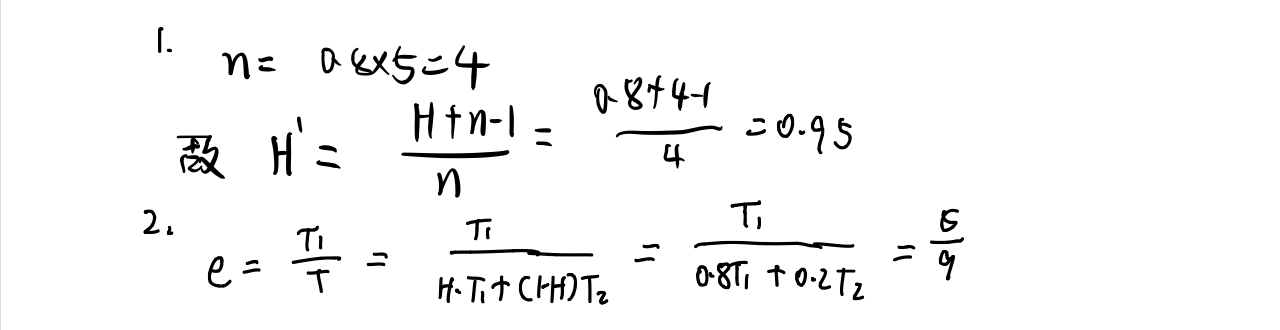## 存储体系原理

### 容量不足的问题

1. 活跃的信息，即当前正在使用的信息
2. 待命的信息，将要使用的信息
3. 静止的信息，已被使用而不再处理的信息

### 速度不足的问题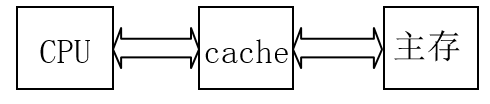### 辅助机构的功能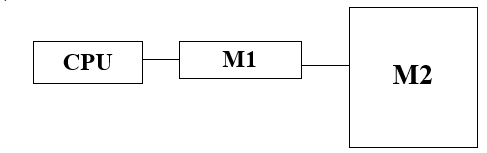$$M_1$$中装满信息的条件下，采用何种算法，算出调用$$M_1$$的部分信息，使$$M_2$$中的部分功能调到$$M_1$$中(即替换算法问题)。

## 存储体系中的页式管理

### 页的定义

页式管理中将虚拟存储空间实际存储空间等分成固定大小的页，使虚拟页可装入主存中不同的实际页面位置。

### 页式管理中的地址表示

页式管理将物理的存储结构进行一层抽象，引入了“页”来进行管理，从而使得对存储地址的访问也需要某种新的地址表示。

1. 虚地址(逻辑地址，程序地址)：包括$$M_2$$在内的大空间地址。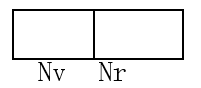$$N_v$$：虚页号

$$N_r$$:页内地址

1. 实地址(物理地址)：为CPU能直接访问的$$M_1$$中的地址。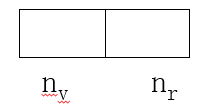$$n_v$$:实页号

$$n_r$$:页内地址

页表具有以下特点：

1. 页表所需行数和虚页号数相等，虚页号与页表行号对应，因此无需虚页号字段。
2. 页表中每行内容分为两个字段：实页号$$n_v$$以及装入位(1位，其中0表示该页已装入，1表示该页未被装入)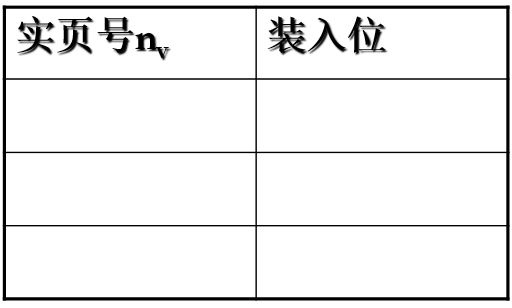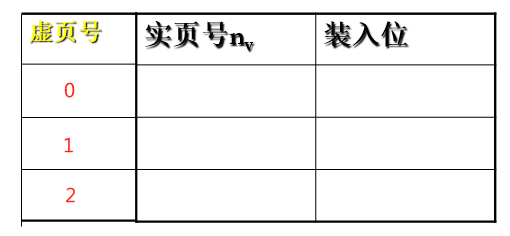### 页式管理的地址变换(关键)

①根据$$N_v$$去查页表中的某一行m。
②查该行的装入位。
③装入位=1时，命中。表示该虚页已装入。

• 从该行中送出$$n_v$$(实页号)。
• 再将$$N_r$$直送$$n_r$$
• 即完成$$N_vN_r$$$$n_v n_r$$

④装入位=0时，失效，表示该虚页未装入$$M_1$$中。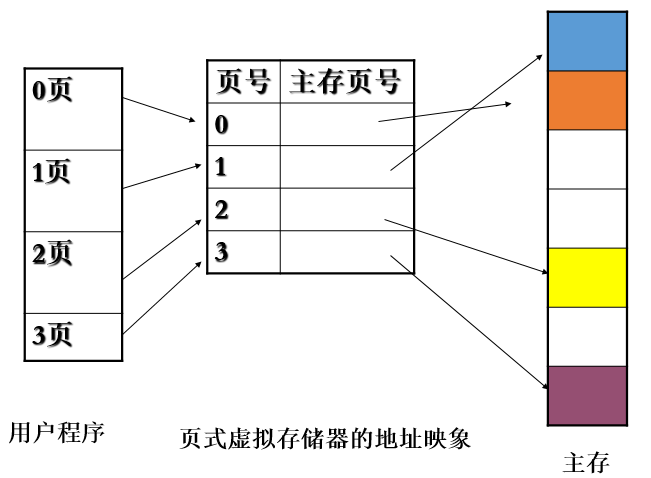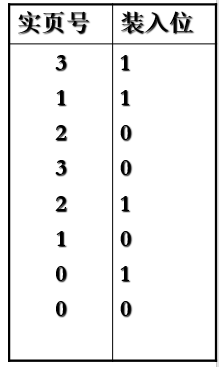1)列出会发生页面失效的全部虚页号；
2)按以下虚地址计算主存实地址：
0、3728、1023、1024、2055、7800、4096、6800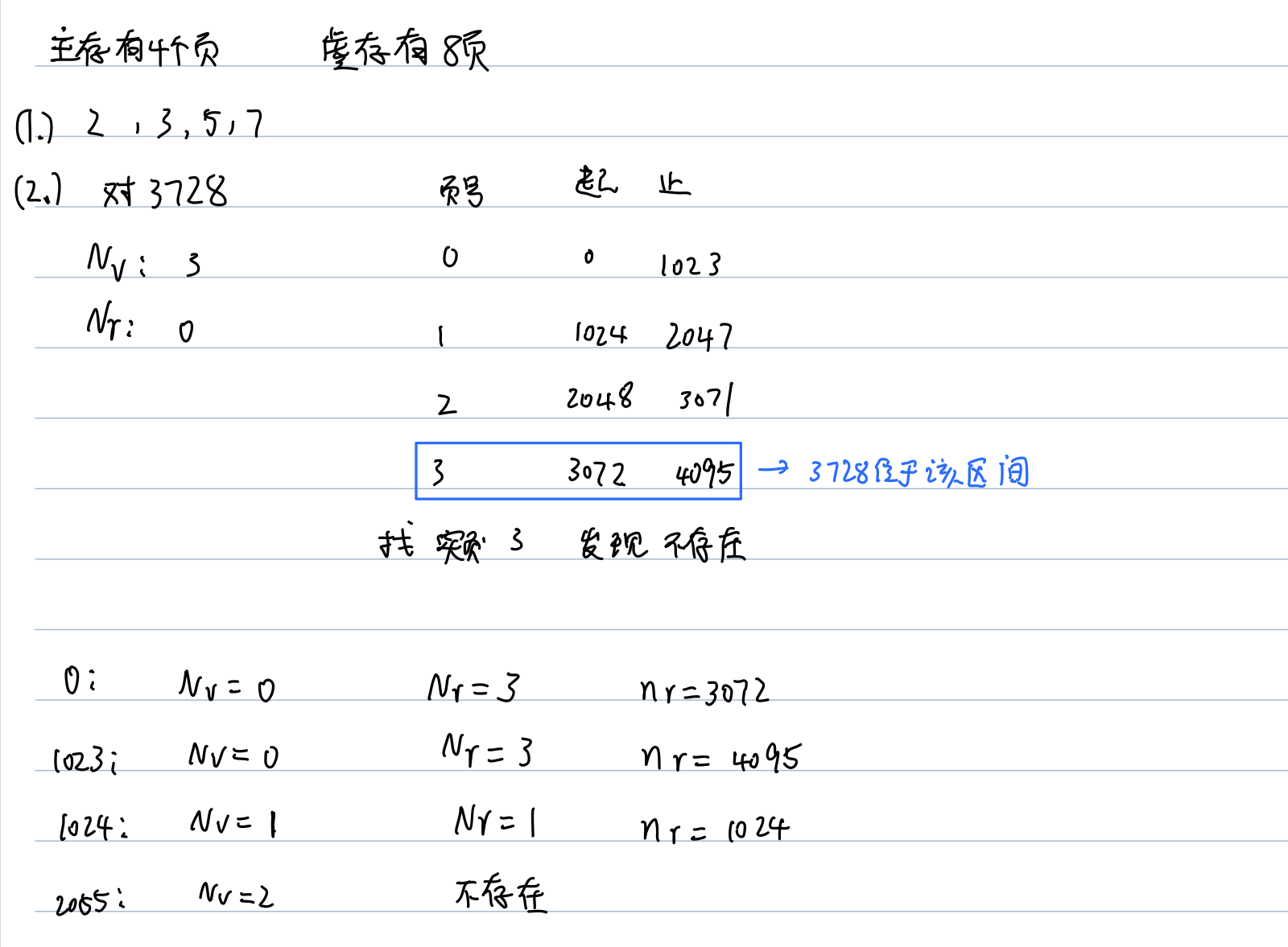## 地址映象及其变换

1. 全相联
2. 直接相联
3. 组相联
4. 段相联

### 全相联映象及其变换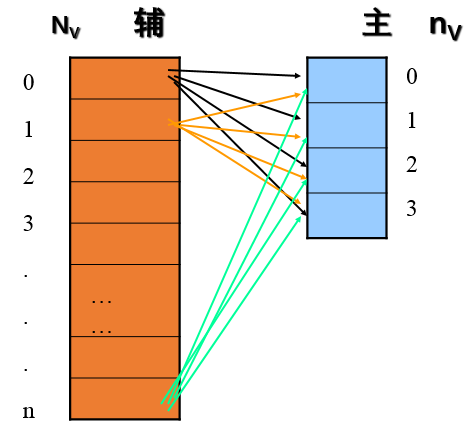#### 地址变换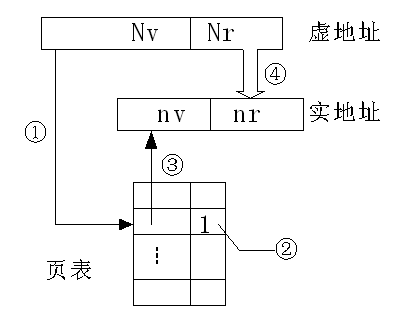#### 全相联目录表法

1. 目录表的行数与主存页面数相等
2. 目录表中每行的内容：
1. $$N_v$$为相联比较字段；
2. $$n_v$$为主存页号(非相联比较字段)。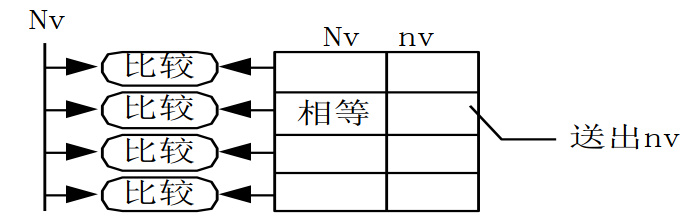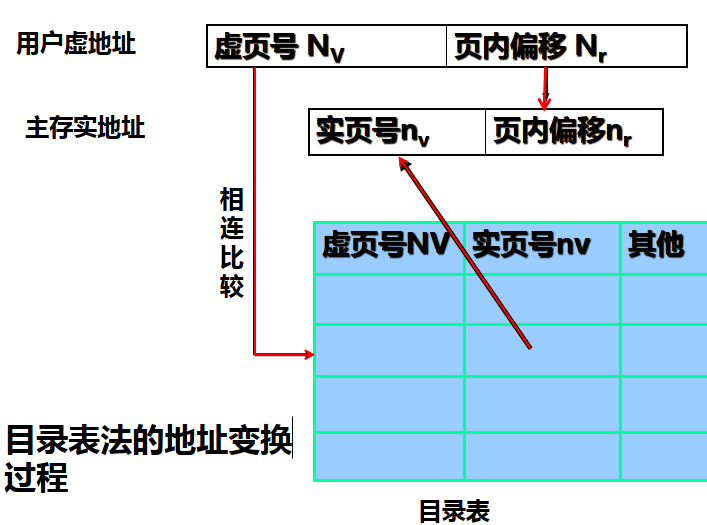1. 将虚地址中的$$N_V$$送目录表中去进行相联比较(一个$$t_m$$)。
2. 当有某个比较器比较相等(比较的时候是比较的是$$N_v$$相关联的字段)时，将该行$$n_V$$送出，同时$$N_r$$$$n_r$$，实现了$$N_vN_r$$$$n_vn_r$$的变换(命中).
3. 若没有相等的，不命中，等待调入。

1. 产生页面冲突的可能性很小(因为页表的行数和主存行数相同)；
2. 与页表放入主存中相比，查表的速度快(因为目录表是放到相联存储器中的，输入高速存储器)；

1. 可扩展性差(因为相联存储器容量在一定程度上限制了主存的扩展，不能太大超过相联存储器的行数)
2. 主存容量增加时，目录表的造价高，速度降低(成本原因，在工程上很常见)。

### 直接映象及其变换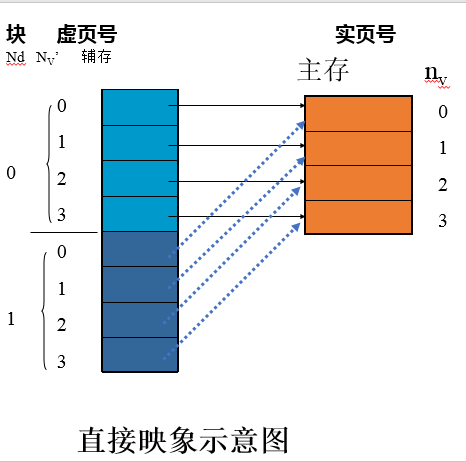• $$Ｎ_d$$ ：块号
• $$N_v′$$：块内页号

#### 地址变换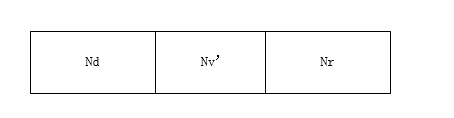块表具有如下特点

1. 块表长度与主存页面数相等。
2. 块表行中的内容：块号$$Ｎ_d$$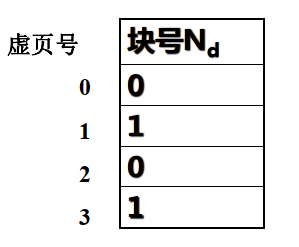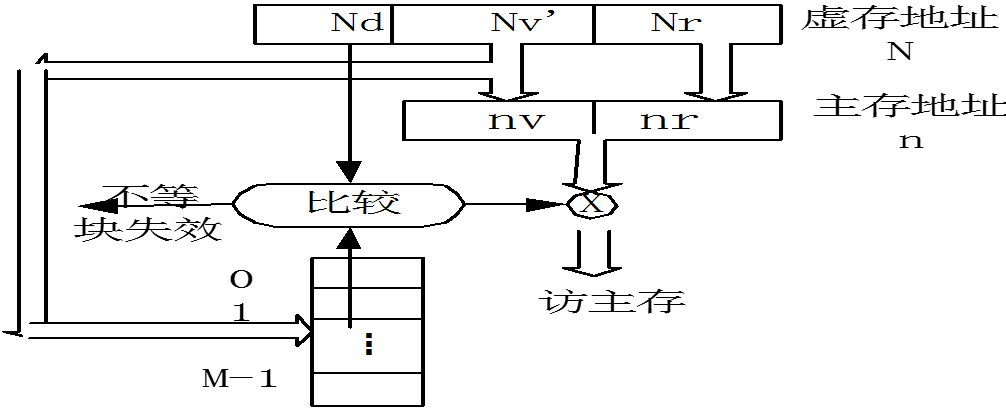1. 根据块内页号$$N_v′$$去查块表中的$$N_v′$$行，获取到对应的块号
2. 将虚地址中的块号$$Ｎ_d$$与所选块表中的 $$Ｎ_d$$比较。
3. 相同时则命中，直接将$$N_v′$$$$n_v$$$$N_r$$$$n_r$$
4. 不同时，则表示未命中

1. 可将查表与访存同时进行，有利于访问速度的提高(命中时)。
2. 产生页面冲突的可能性极大(因无灵活的存放余地)。

### 组相联映象及地址变换

组相连映像则是先将主存分为页面数相同的若干组，再将辅存按主存划分为若干区，组内采用全相联映象，组间采用直接映象。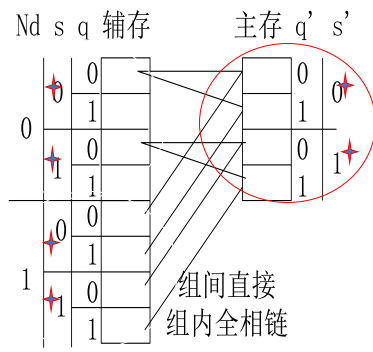• $$Nd$$:区号
• $$s$$ :组号
• $$q$$:组内页号——辅存
• $$s'$$:组号
• $$q′$$组内页号——主存

#### 地址变换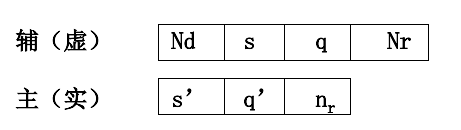1. 表的行数与组数相等(本例2组，即2行)。
2. 每 行 大 字 段 数与组内页面数相等(本例2个 )。
3. 每 个 大 字 段 又分为三个小字段。
4. 每个大字段还有一个比较器。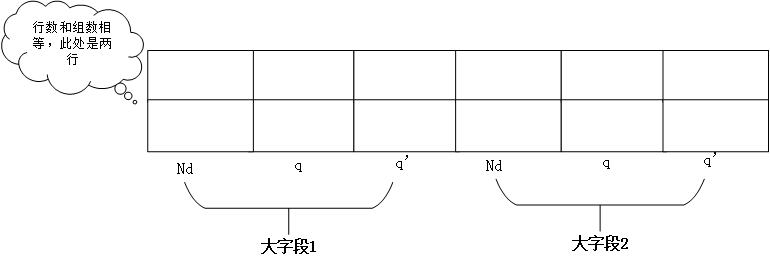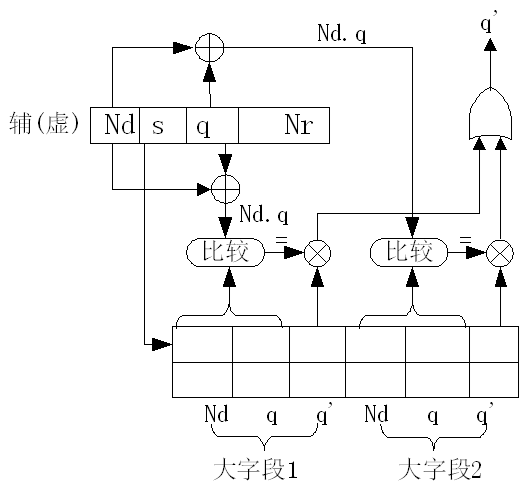1. 根据虚地址中的s去查RAM表中的某一行。
2. 将虚地址中的$$N_d$$、q同时送各比较器与所选行中的$$N_d$$、q进行比较。
3. 当有一个比较器相等时：
1. 将s→s’(组间直接)。
2. 将相等大字段中q’送出作q’。
3. 再将$$N_r$$$$n_r$$,即实现了将虚址($$N_d$$,s ,q ,$$N_r$$ )→( s′, q′,$$n_r$$)命中时的地址变换

### 段相联映象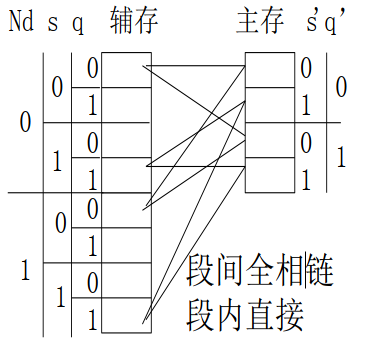## 四种映象规则的关系

1. 在组相联映象中，当每组只有一页时，此时的组相联就是直接映象。

当把主存只分为一个组时，此时的组相联也就是全相联映象，即直接映象和全相联映象是组相联映象的两个特例。

2. 在段相联映象中，当每段只有一页时，此时的段相联映象就是全相联映象。

当把主存只分为一个段时，此时的段相联也就是直接映象。

## 总结

06-081129
03-02702
12-011584
01-056489
04-09
05-132万+
08-20
05-10311
05-045885
01-102307
10-25869
08-18712
05-23997
11-17173

### “相关推荐”对你有帮助么？

•非常没帮助
•没帮助
•一般
•有帮助
•非常有帮助被折叠的  条评论 为什么被折叠?到【灌水乐园】发言vcjmhg

¥1 ¥2 ¥4 ¥6 ¥10 ¥20获取中扫码支付点击重新获取扫码支付1.余额是钱包充值的虚拟货币，按照1:1的比例进行支付金额的抵扣。
2.余额无法直接购买下载，可以购买VIP、付费专栏及课程。余额充值